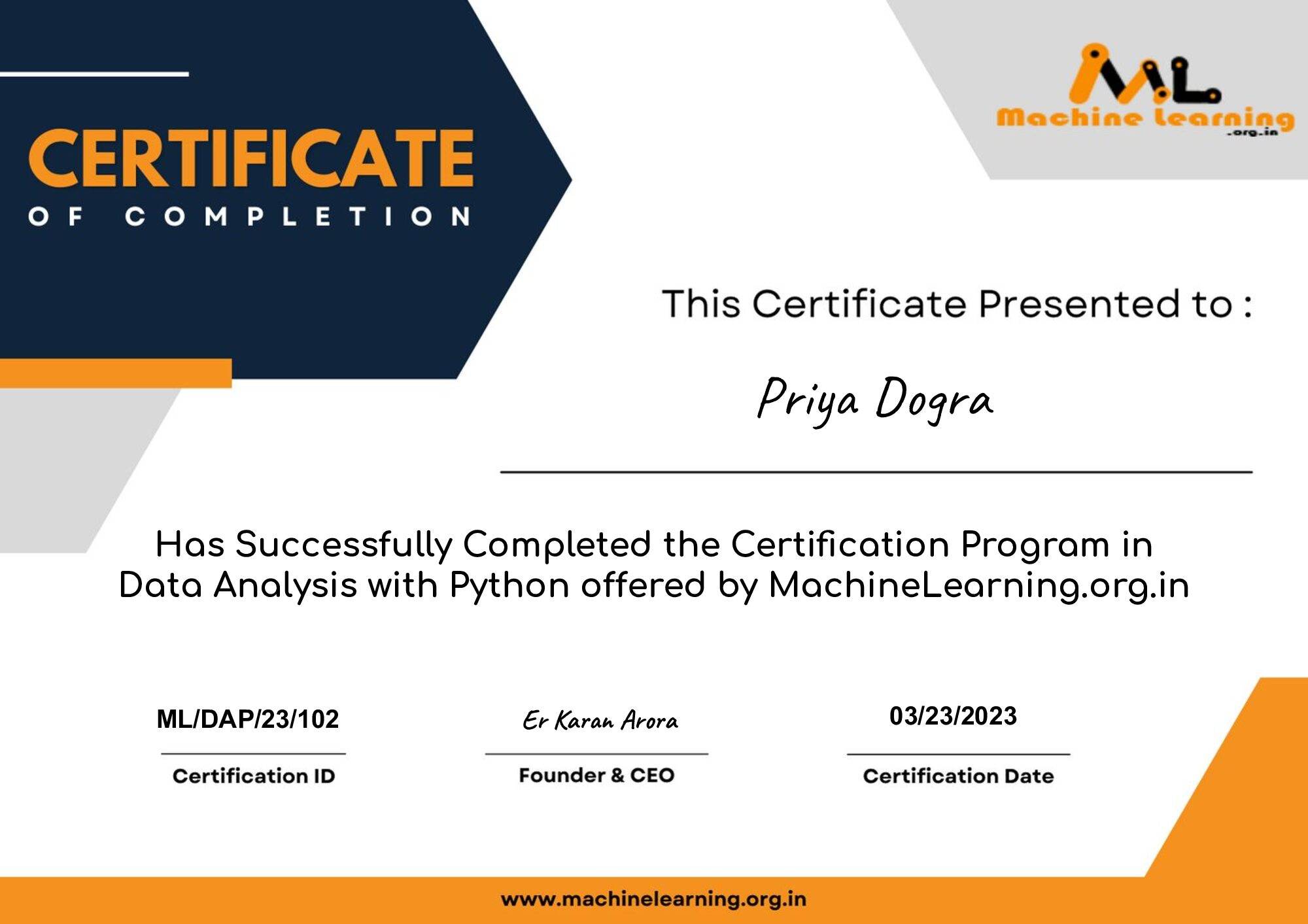Home Quiz Data Analysis with Python Free Certification – Machinelearning.org.in

# Data Analysis with Python Free Certification – Machinelearning.org.in

0Get Data Analysis with Python Certificate from  MachineLearning.org.in which you can share in the Certifications section of your LinkedIn profile, on printed resumes, CVs, or other documents.

Exam Details

• Format: Multiple Choice Question
• Questions: 10
• Passing Score: 8/10 or 80%
• Language: English

Here are the questions and answers :

What does CSV stand for?

Comma-separated values

Car sold values
Car state values
None of the above

What is the command to display the first five rows of a dataframe df?

df.tail()
df.first()
df.first(5)

Consider the dataframe df. What does the command df.rename(columns={‘a’:’b’}) change about the dataframe df?

Renames column “a” of the dataframe to “b”.
Renames row “a” to “b”.
Nothing. You must set the parameter “inplace = True”.
replace a with b in entire column

Consider the column of the dataframe, df[‘Fuel’], with two values: ‘gas’ and’ diesel’. What will be the name of the new columns pd.get_dumies(df[‘Fuel’]) ?

1 and 0
Just ‘diesel’
Just ‘gas’
‘gas’ and ‘diesel’

Consider the dataframe “df”. What method provides the summary statistics?

df.describe()
df.tail()
df.info()

What value of R^2 (coefficient of determination) indicates your model performs best?

-100
-1
0
1

Which statement is true about polynomial linear regression?

Polynomial linear regression is not linear in any way.
Although the predictor variables of polynomial linear regression are not linear, the relationship between the parameters or coefficients is linear.
Polynomial linear regression uses wavelets.
all of the above

We create a polynomial feature PolynomialFeatures(degree=2). What is the order of the polynomial?

0
1
2
3

What does K stand for in K mean algorithm?

number of clusters
number of data
number of attributes
Number of iterations

Identify the revision control system on the following.

Scipy
Numpy
Git
Scikit-Learn